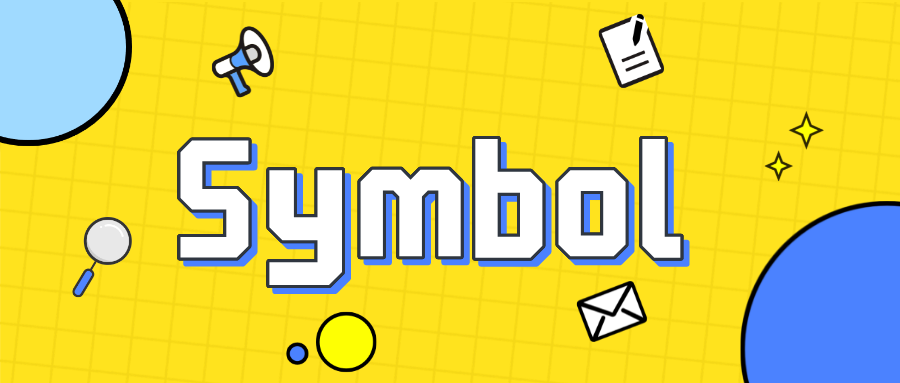# Symbol 需要知道的知识点## 是什么？

Symbol 是 ES6 新增的基本数据类型–符号，它具有唯一性、不可变性。因此能确保对象属性的唯一性，不会发生冲突。

Symbol 和其他基本类型：null、undefined、boolean、number、string的不同是没有对应的包装类和 new 一起使用。

``````let s = new String('zhangsan')
``````

``````let s = new Symbol()
// Uncaught TypeError: Symbol is not a constructor
``````

### 基本用法

``````let s = Symbol()
let name = Symbol('name') // 传入字符串作为符号的描述，主要用于调试代码
``````

``````let s1 = Symbol()
let s2 = Symbol()

s1 == s2 // false

let s3 = Symbol('name')
let s4 = Symbol('name')

s3 == s4 // false
``````

``````let name = Symbol('name')
let o = {
[name]:'zhangsan'
}

// or

let name = Symbol('name')
let o = {}
o[name] = 'zhangsan'
``````

``````let id = Symbol("id");

people[id] = "新增值";
``````

### 全局符号注册表

Symbol 每次创建都是唯一的，那如何复用呢？ Symbol.for 就解决了共享和重用问题。

``````let name = Symbol.for('name') // 第一次时全局注册表不存在则创建并添加到注册表中。

let otherName = Symbol.for('name') // 后续使用相同字符串，先检索全局注册表有就返回，反之创建。

name == otherName // true
``````

``````let name = Symbol.for('name')
Symbol.keyFor(name) // 'name'
``````

``````let name = Symbol('name')
Symbol.keyFor(name) // undefined
``````

### 对象属性遍历

for…in 会忽略 Symbol：

``````let id = Symbol("id");
let user = {
name: "John",
age: 30,
[id]: 123
};

for (let key in user) alert(key); // name, age (no symbols)
Object.keys(usr) // ['name','age']
Object.getOwnPropertyNames(user) // ['name','age']
Object.getOwnPropertySymbols(user) // [Symbol(id)]

let clone = Object.assign({}, user);
clone[id] // 123

``````